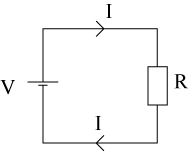#### You may also like### On the Road

Four vehicles travelled on a road. What can you deduce from the times that they met?### Intersections

Change one equation in this pair of simultaneous equations very slightly and there is a big change in the solution. Why?### Perpendicular Lines

Position the lines so that they are perpendicular to each other. What can you say about the equations of perpendicular lines?

# Electric Kettle

##### Age 14 to 16Challenge Level

The diagram shows a simple circuit: the cell provides energy, V volts, which causes a current, I amps, to flow around the circuit. There is also a resistance, R ohms.This circuit provides a simple model for what happens in an electric kettle: a resistance converts electrical energy into heat energy by impeding the flow of electrons around the circuit.

The table shows data collected from a circuit like this.

 Resistance (ohms) Temperature (degrees Celsius) 5 44.9 6 50 7 55.1 8 59.9 9 65 10 70.1

• Draw a graph of this data, with the resistance on the horizontal axis, putting a straight line through the points.
• Find the gradient of the line.
• Find the equation of the line.

Once you have found the equation, discuss these questions:

• What resistance would you need to heat water to 100 C °?
• What would the temperature be if the resistance was zero?
• Do you think that in practice, any circuit can have zero resistance?